Hits: 1

# Complete Binary Tree

#### In this tutorial, you will learn about a complete binary tree and its different types. Also, you will find working examples of a complete binary tree in Python.

A complete binary tree is a binary tree in which all the levels are completely filled except possibly the lowest one, which is filled from the left.

A complete binary tree is just like a full binary tree, but with two major differences

1. All the leaf elements must lean towards the left.
2. The last leaf element might not have a right sibling i.e. a complete binary tree doesn’t have to be a full binary tree.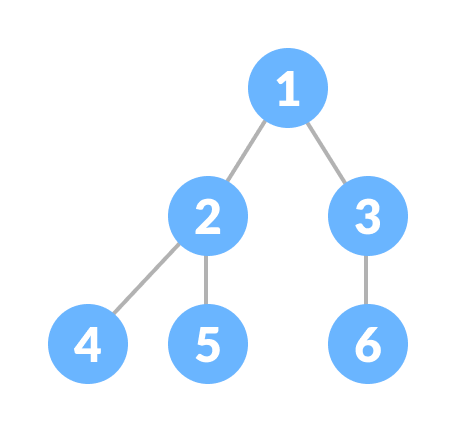Complete Binary Tree

## Full Binary Tree vs Complete Binary Tree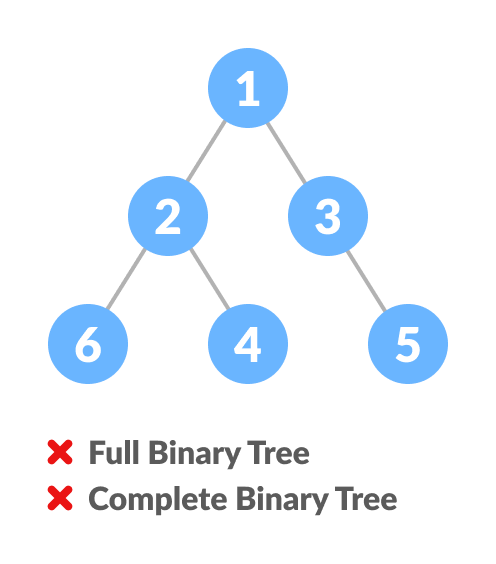Comparison between full binary tree and complete binary tree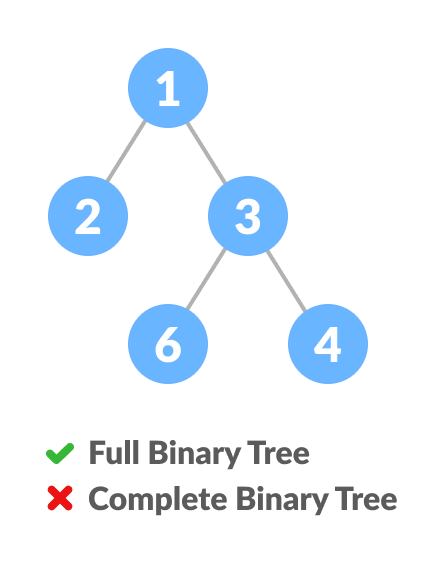Comparison between full binary tree and complete binary tree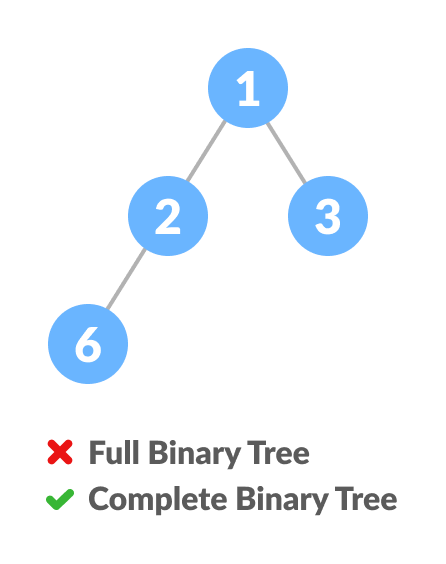Comparison between full binary tree and complete binary tree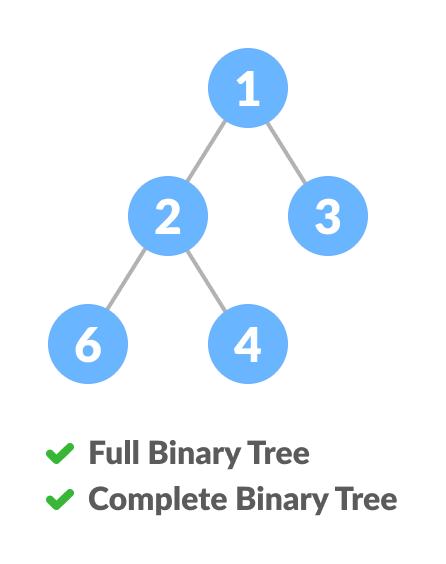Comparison between full binary tree and complete binary tree

## How a Complete Binary Tree is Created?

1. Select the first element of the list to be the root node. (no. of elements on level-I: 1)Select the first element as root
2. Put the second element as a left child of the root node and the third element as the right child. (no. of elements on level-II: 2)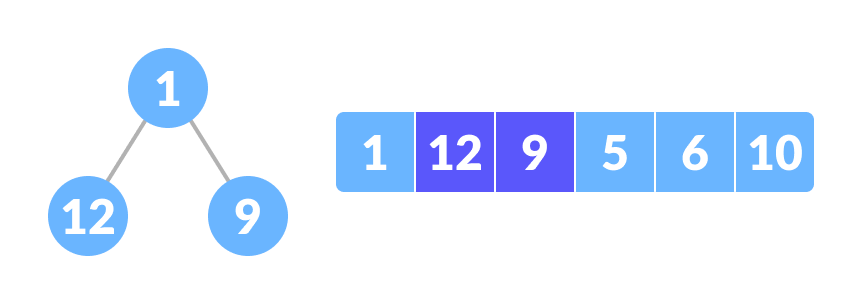12 as a left child and 9 as a right child
3. Put the next two elements as children of the left node of the second level. Again, put the next two elements as children of the right node of the second level (no. of elements on level-III: 4) elements).
4. Keep repeating until you reach the last element.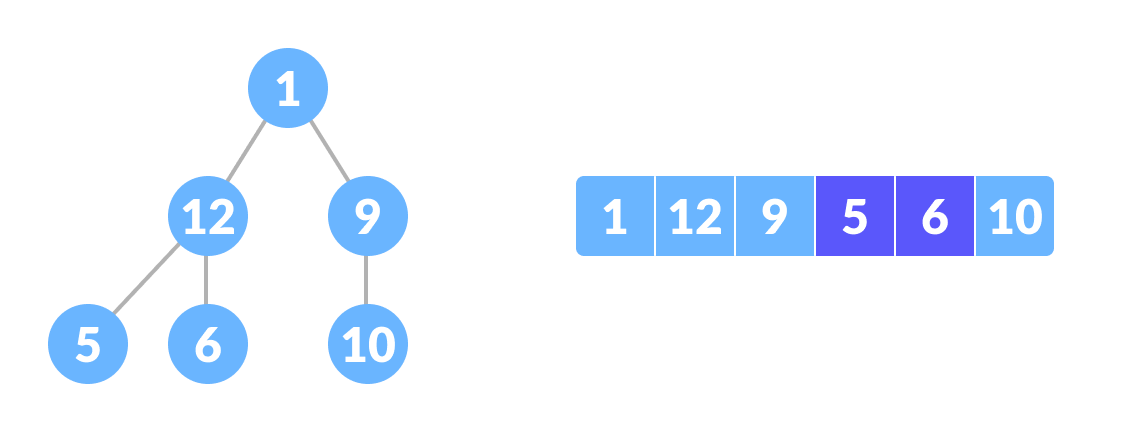5 as a left child and 6 as a right child

## Python Examples

``````/* Checking if a binary tree is a complete binary tree in Python */

class Node:

def __init__(self, item):
self.item = item
self.left = None
self.right = None

/* Count the number of nodes */
def count_nodes(root):
if root is None:
return 0
return (1 + count_nodes(root.left) + count_nodes(root.right))

/* Check if the tree is complete binary tree */
def is_complete(root, index, numberNodes):

/* Check if the tree is empty */
if root is None:
return True

if index >= numberNodes:
return False

return (is_complete(root.left, 2 * index + 1, numberNodes)
and is_complete(root.right, 2 * index + 2, numberNodes))

root = Node(1)
root.left = Node(2)
root.right = Node(3)
root.left.left = Node(4)
root.left.right = Node(5)
root.right.left = Node(6)

node_count = count_nodes(root)
index = 0

if is_complete(root, index, node_count):
print("The tree is a complete binary tree")
else:
print("The tree is not a complete binary tree")
``````

## Relationship between array indexes and tree element

A complete binary tree has an interesting property that we can use to find the children and parents of any node.

If the index of any element in the array is i, the element in the index `2i+1` will become the left child and element in `2i+2` index will become the right child. Also, the parent of any element at index i is given by the lower bound of `(i-1)/2`.

Let’s test it out,

```Left child of 1 (index 0)
= element in (2*0+1) index
= element in 1 index
= 12

Right child of 1
= element in (2*0+2) index
= element in 2 index
= 9

Similarly,
Left child of 12 (index 1)
= element in (2*1+1) index
= element in 3 index
= 5

Right child of 12
= element in (2*1+2) index
= element in 4 index
= 6

```

Let us also confirm that the rules hold for finding parent of any node

```Parent of 9 (position 2)
= (2-1)/2
= ½
= 0.5
~ 0 index
= 1

Parent of 12 (position 1)
= (1-1)/2
= 0 index
= 1
```

Understanding this mapping of array indexes to tree positions is critical to understanding how the Heap Data Structure works and how it is used to implement Heap Sort.

# Special 95% discount

## 2000+ Applied Machine Learning & Data Science Recipes

### Portfolio Projects for Aspiring Data Scientists: Tabular Text & Image Data Analytics as well as Time Series Forecasting in Python & R## Two Machine Learning Fields

There are two sides to machine learning:

• Practical Machine Learning:This is about querying databases, cleaning data, writing scripts to transform data and gluing algorithm and libraries together and writing custom code to squeeze reliable answers from data to satisfy difficult and ill defined questions. It’s the mess of reality.
• Theoretical Machine Learning: This is about math and abstraction and idealized scenarios and limits and beauty and informing what is possible. It is a whole lot neater and cleaner and removed from the mess of reality.

Data Science Resources: Data Science Recipes and Applied Machine Learning Recipes

Introduction to Applied Machine Learning & Data Science for Beginners, Business Analysts, Students, Researchers and Freelancers with Python & R Codes @ Western Australian Center for Applied Machine Learning & Data Science (WACAMLDS) !!!

Latest end-to-end Learn by Coding Recipes in Project-Based Learning:

Applied Statistics with R for Beginners and Business Professionals

Data Science and Machine Learning Projects in Python: Tabular Data Analytics

Data Science and Machine Learning Projects in R: Tabular Data Analytics

Python Machine Learning & Data Science Recipes: Learn by Coding

R Machine Learning & Data Science Recipes: Learn by Coding

Comparing Different Machine Learning Algorithms in Python for Classification (FREE)

`Disclaimer: The information and code presented within this recipe/tutorial is only for educational and coaching purposes for beginners and developers. Anyone can practice and apply the recipe/tutorial presented here, but the reader is taking full responsibility for his/her actions. The author (content curator) of this recipe (code / program) has made every effort to ensure the accuracy of the information was correct at time of publication. The author (content curator) does not assume and hereby disclaims any liability to any party for any loss, damage, or disruption caused by errors or omissions, whether such errors or omissions result from accident, negligence, or any other cause. The information presented here could also be found in public knowledge domains.  `